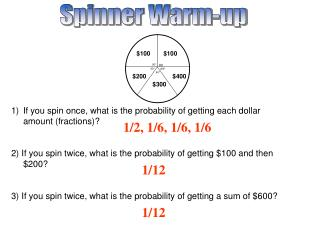DownloadDownload PresentationSpinner Warm-up

# Spinner Warm-up

Download Presentation## Spinner Warm-up

- - - - - - - - - - - - - - - - - - - - - - - - - - - E N D - - - - - - - - - - - - - - - - - - - - - - - - - - -
##### Presentation Transcript

1. Spinner Warm-up \$100 \$100 90° 90° 60° 60° 60° \$200 \$400 \$300 • If you spin once, what is the probability of getting each dollar amount (fractions)? • 2) If you spin twice, what is the probability of getting \$100 and then \$200? • 3) If you spin twice, what is the probability of getting a sum of \$600? 1/2, 1/6, 1/6, 1/6 1/12 1/12

2. More Warm up. = 2.35 Use your calculator to find the decimal answer. Round to the nearest hundred = 0.13

3. Use the table of values to determine the function represented. Benchmark Question #1 c • g(x) = x b) g(x) = x2 • c) g(x) = IxI d) g(x) = x3

4. In Triangle ABC, AC=6, AB=7, and BC=5. Which of the following statements is true? Benchmark Question #2 • The measure of angle C is the least of the three angles. • The measure of angle C is the greatest of the three angles • The measure of angle B is the greatest of the three angles • The measure of angle B is the least of the three angles b

5. Based on the graph of the following function, what is the greatest rate of decrease for the function? Benchmark Question #3 • -4 • -10/3 • -7/2 • -5/3 c

6. Benchmark Question #4 Add the following. d

7. GPS ALGEBRA UNIT QUESTION: How do you use probability to make plans and predict for the future? Standard: MM1D1-3 Today’s Question: When do you find the expected value of an experiment? Standard: MM1D2.d.

8. Expected Value • A collection of outcomes is partitioned into n events, no two of which have any outcomes in common. The probabilities of the n events occurring are p1, p2, p3,..., pn where p1 + p2 + p3 + pn = 1. The values of the n events are x1, x2, x3,..., xn. • E = p1x1 + p2x2 + p3x3 + ... + pnxn

9. Example 1 • Find the expected value. E = 1(.20) + 2(.30) + 3(.10) + 4(.40) = 2.7 If I do this experiment 10 times, what total value would you expect to get?

10. Example 2 • You take an exam that has 4 possible answers for each question. You gain 3 points for each correct answer, lose 1 point for each incorrect answer, and do not gain or lose points for blank answers. If you guess on a question, what is the expected value for the number of points you receive? E = (3)(1/4) + (-1)(3/4) = 0

11. Example 3 • At a raffle, 2500 tickets are sold at \$5 each for 3 prizes of \$1000, \$500, and \$100. You buy one ticket. What is the expected value of your winnings? E = 995(1/2500) + 495(1/2500) + 95(1/2500) + (-5)(2497/2500) = \$ –4.36

12. Classwork Workbook (pages 375-376)